Homework Help Question & Answers

# The density of mercury is 13.6 g/cm 3. What is the density of mercury in kg/m...

The density of mercury is 13.6 g/cm 3. What is the density of mercury in kg/m 3? Show work please

#### Homework Answers

Answer #1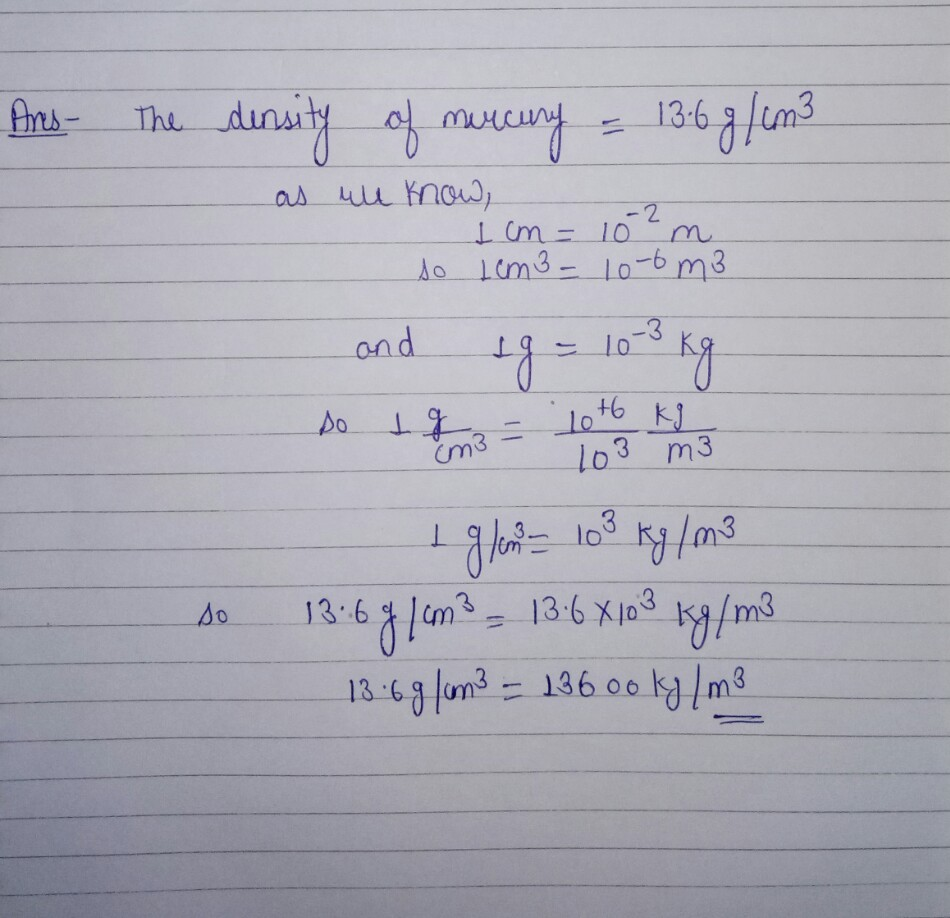Know the answer?
Your Answer:

#### Post as a guest

Your Name:

What's your source?

#### Earn Coin

Coins can be redeemed for fabulous gifts.

Not the answer you're looking for? Ask your own homework help question. Our experts will answer your question WITHIN MINUTES for Free.
Similar Homework Help Questions
• ### In the figure, if the tube is filled with mercury of density 13.6*10^3 and the level...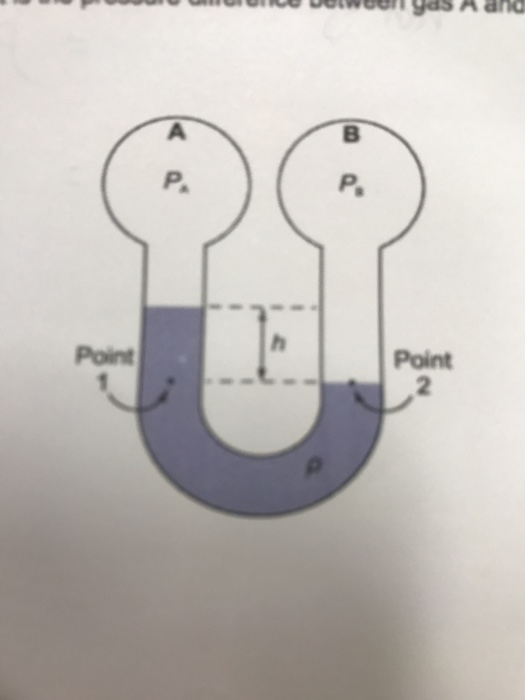In the figure, if the tube is filled with mercury of density 13.6*10^3 and the level difference is(h=10 cm) what is the pressure difference between gas A and B A ano P. Point Point 2

• ### 6) The density of water is 1.0 g/cm and the density of Hg is 13.6 g/cm'....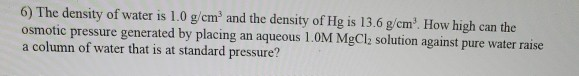6) The density of water is 1.0 g/cm and the density of Hg is 13.6 g/cm'. How high can the osmotic pressure generated by placing an aqueous 1.OM MgCl, solution against pure water raise a column of water that is at standard pressure?

• ### please help me in this A mercury height of 28 cm is equivalent to a height...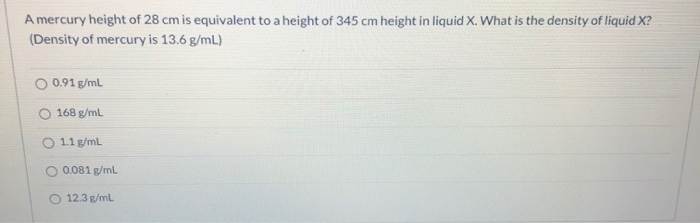please help me in this A mercury height of 28 cm is equivalent to a height of 345 cm height in liquid X. What is the density of liquid X? (Density of mercury is 13.6 g/mL) O 0.91 g/ml 168 g/mL 1.1 g/mL O 0.081 g/mL 12.3 g/mL

• ### 20) The density of a block of iron is 7,860 kg/m². The density of mercury is...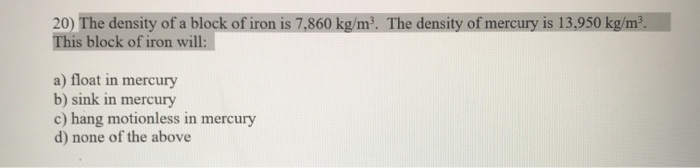20) The density of a block of iron is 7,860 kg/m². The density of mercury is 13,950 kg/m² This block of iron will: a) float in mercury b) sink in mercury c) hang motionless in mercury d) none of the above

• ### What pressure (in atm) is exerted by a column of mercury 1.40 m high? The density...

What pressure (in atm) is exerted by a column of mercury 1.40 m high? The density of mercury is 13.5951 g/cm^3.

• ### 7. (20 pts) Mercury (density 13500 kg/m) is poured into a large beaker to a depth...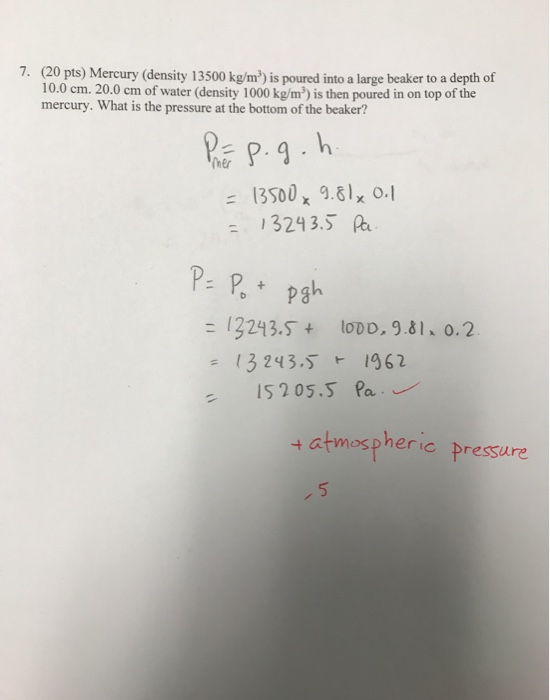7. (20 pts) Mercury (density 13500 kg/m) is poured into a large beaker to a depth of 10.0 cm. 20.0 cm of water (density 1000 kg/m) is then poured in on top of the mercury. What is the pressure at the bottom of the beaker? Pap.g.h. = 13500x9.8lx 0.1 = 13243.5 Pa. P = Pot pgh = 12243.5+ 100.9.81.0.2. = 13243.5 * 1962 - 15205.5 Pa.. + atmospheric pressure -5

• ### A 76.00 pound flask of mercury costs \$154.00. The density of mercury is 13.534 g/cm'. Find...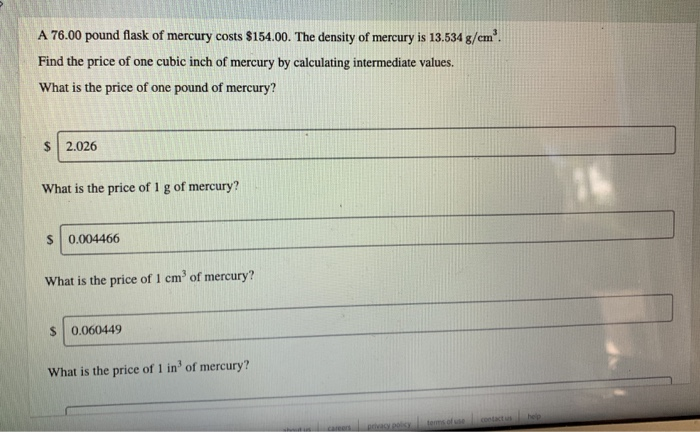A 76.00 pound flask of mercury costs \$154.00. The density of mercury is 13.534 g/cm'. Find the price of one cubic inch of mercury by calculating intermediate values. What is the price of one pound of mercury? \$ 2.026 What is the price of 1 g of mercury? 0.004466 What is the price of 1 cm of mercury? \$ 0.060449 What is the price of 1 in of mercury? What is the price of 1 in of mercury? \$ 1...

• ### if the density of mercury is 13.8g/cm3. calculate the mass in kg of 2.0L of mercury...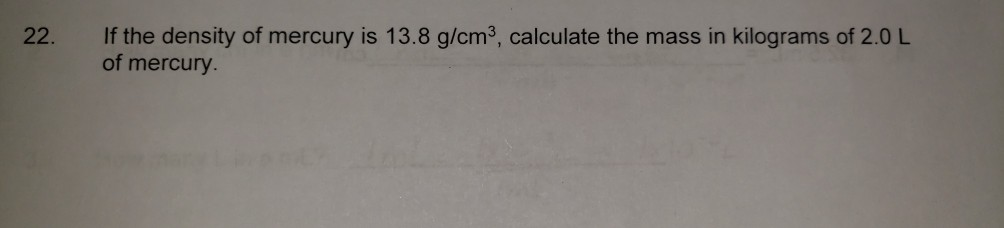if the density of mercury is 13.8g/cm3. calculate the mass in kg of 2.0L of mercury If the density of mercury is 13.8 g/cm3, calculate the mass in kilograms of 2.0 L of mercury.

• ### Calculate the mass density of the following string: m=35.0 g, L=75 cm, mass density=? kg/m If...

Calculate the mass density of the following string: m=35.0 g, L=75 cm, mass density=? kg/m If a string with this mass density is under 100 N, then the speed of any wave would be: v=? m/s

• ### Convert: 2.7x10^-3 kg/cm^3 to kg/m^3 0.81 g/cm^3 to kg/m^3

Convert:2.7x10^-3 kg/cm^3 to kg/m^30.81 g/cm^3 to kg/m^3

Free Homework App

Scan Your Homework
to Get Instant Free Answers
Need Online Homework Help?

Get Answers For Free
Most questions answered within 3 hours.